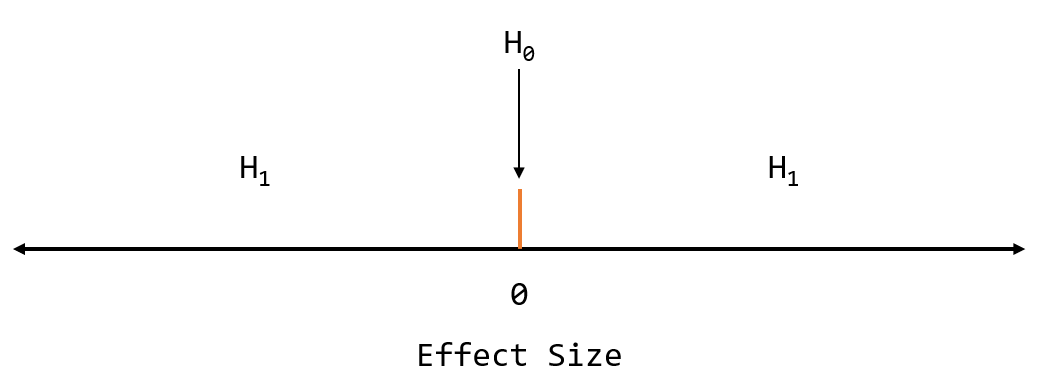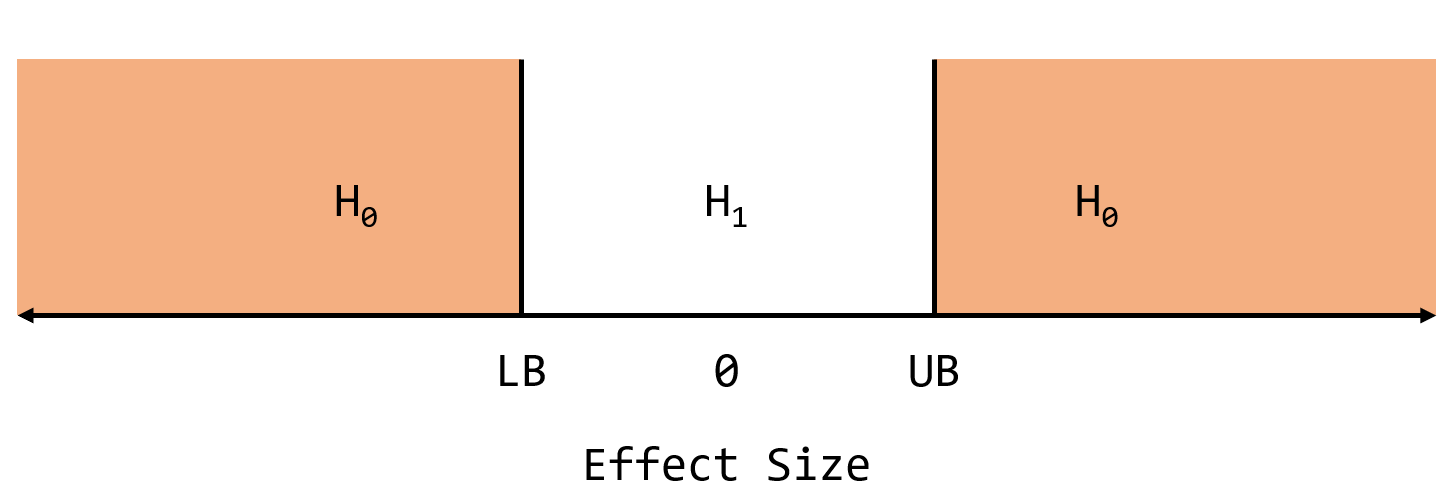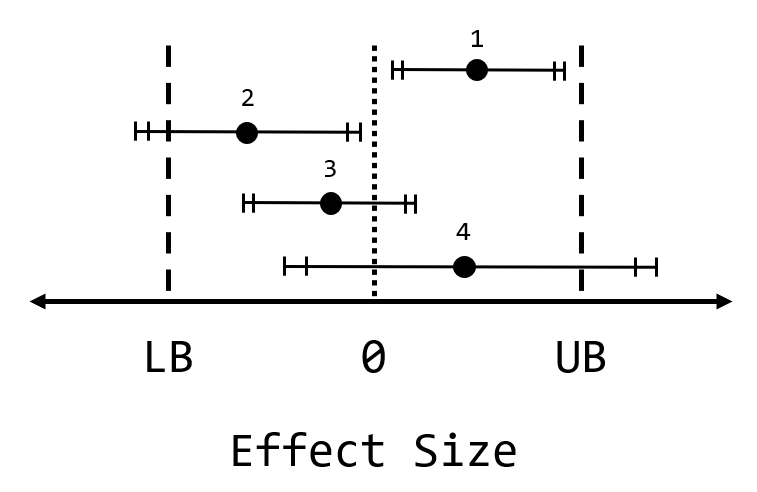# Testing for no effect

A/B testing is the process of running an experiment on your user base in order to determine whether a particular change in your product (or service) had the expected effect on some metric that you’re concerned with. It helps you quantify whether the change you made actually had an effect on your users’ behaviour, and thus helps answer questions like:

• Did the change we made in how search results are presented improve click-through rates?
• Did improvements in page load speed decrease the bounce rate?
• Did changing the colour of the button really affect user engagement?

A/B testing is widely used in the industry and is one reason, I believe, that the big tech companies are so good at driving engagement. (Whether the right sort of engagement is being driven is another topic altogether.)

But what if you want to ensure that a change you made did not impact user behaviour? What tools do we have for that?

## A/B testing: A background

The predominant form of A/B testing appears to be the Null Hypothesis Significant Test (NHST). In such a statistical test, you start out with the assumption (or the null hypothesis, labeled as $$H_0)$$ that there is no difference between your control and treatment groups. (The treatment group being the one that gets your changed experience, while the control group gets the baseline or current experience) You then set out to disprove this assumption or hypothesis by collecting data (a sample) on the two groups to determine how they differed across some metric of concern.

The basic idea is that you calculate a p-value (from an appropriate test statistic calculated from the observed data in your sample) and use this p-value to guide your behaviour. The p-value is the likelihood of observing a result at least as extreme as the one in your observed data, assuming the null hypothesis is true, i.e. assuming there is no difference between the groups. The exact definition is:

p-value: The probability of obtaining a result at least as extreme as the actual observed results, given that the null hypothesis is true.

If the p-value is below some threshold, you conclude that the observed data are unlikely to have been produced assuming the null hypothesis is true, so you act as if the null hypothesis is false, or you reject the null hypothesis and basically conclude that there is a difference between the groups. Thus a p-value below the threshold is considered support for the alternative hypothesis, or $$H_1$$, that there is a difference between the groups. This process is akin to a probabilistic proof by contradiction argument: Show that the observed data are inconsistent with the assumption that the control and treatment groups are the same.

The p-value threshold used for your decision is labeled as $$\alpha$$ and is known as the significance level, and typical values are 0.05 or 0.01, but you can set it to whatever you want. $$\alpha$$ acts as a control on your long-term Type 1 or false positive error rate. For more on NHST, I recommend this great visualization by R Psychologist.

Assuming that your control and treatment groups were created in such a way that there was no systemic difference between them (other than the change you are testing), you can further conclude that the observed difference was associated with your change.

You also typically obtain an estimate of the true difference by calculating the difference between the two groups and also producing a confidence interval around that estimate which describes the uncertainty in the estimate.

It’s important to properly interpret a p-value. Because NHST is a Frequentist approach, the concepts tend to be unintuitive, or at least less intuitive than Bayesian concepts. In particular, the p-value is not the probability that the null hypothesis is true, nor is it the probability that you have a false positive. The p-value is instead a conditional probability of roughly the form:

$$p = P(D \geq d|H_0)$$

where

• $$D \geq d$$: Observing a difference at least as large as the observed difference, $$d$$
(This is for a one-sided test)

One consequence of how the p-value is formulated here is that it’s not straightforward to prove that there is not a difference. This is because the p-value is a conditional probability given the null hypothesis $$H_0$$ is true. The p-value makes no direct statement as to whether $$H_0$$ or $$H_1$$ is true or false.

We haven’t computed any direct probability on $$H_0$$, and furthermore, when $$p > \alpha$$ the only correct conclusion is that the observed data were not surprising, assuming there was no difference between the groups.

Thus, in general, if you do a test and get a high p-value, it’s not correct to conclude that there is no difference between the groups purely based on the p-value alone. In another words, failing to reject the null is not strong support for the null. This point is further emphasized when you consider that p-values under the null hypothesis are uniformly distributed, that is, you’re just as likely to get values between [0, 0.05] as you are between [0.95, 1.00] when there is no difference between the groups.

This is one of the drawbacks of this form of NHST based purely off p-values: While it you can reject the null, you cannot provide support for it. That is, you cannot provide support for the absence of a difference. In other words, absence of evidence is not evidence of absence. (This is a well-known problem in the philosophy of science.)

So how can we properly do an A/A test then?

## A/A testing and Equivalence Testing

A/A testing, that is, testing for the absence of any meaningful change in user behaviour after making some change, is less common than A/B testing but is still useful. Here are some potential situations where an A/A test would be helpful:

• You have to do a complex migration from one backend system to another that should not affect how your website behaves to end users.
• You want to remove some functionality from your application that you believe hardly anyone is using, and thus don’t expect a change in engagement beyond -X %
• You want to replace a ranking model with a less complicated model that is faster and that performed equally well when tested on historical data. You therefore don’t expect a change in metrics beyond a certain range.

An Equivalence Test is type of hypothesis test that can provide better statistical support for these types of experiments than a traditional NHST. It is a way to formally test for the lack of a meaningful effect while controlling your long-term error rates. By contrast, using a traditional NHST to check for the lack of a meaningful effect size is an informal ad-hoc approach that doesn’t provide a way to control for error rates.

In equivalence testing the test setup is the same: You create your control (baseline experience) and treatment (new experience) groups, run the experiment, and collect the necessary data. The only change is in how the null hypothesis is defined, and hence how the test statistics are calculated.

In an equivalence test, the null hypothesis is instead defined to be:

$$H_0$$: There is a difference between the two groups outside of some set lower and upper bounds.

It’s up to you to define what the lower and upper bounds should be, but they should reflect the range for an effect that you consider to be trivial or not meaningful. This range then becomes your equivalence bounds: The range of effect sizes that don’t matter to you.

It’s best to visualize this in comparison to the null for traditional NHST.

In traditional NHST the null hypothesis is that the effect size is exactly zero, i.e. that there is no difference between the groups, and the alternative hypothesis is that the effect size is non-zero:For an equivalence test, the null hypothesis is that there is an effect size of less than the lower bound (LB) or more than the upper bound (UB). By contrast, the alternative hypothesis is that the effect size is between the lower and upper bounds:We then set out to disprove this null hypothesis, as was the case for traditional NHST. If we can reject this null, then we can act as if the true effect size is within the equivalence bounds and hence the effect size difference between the two groups is statistically equivalent. This ultimately provides support for what we wanted: That there was no difference in user behaviour introduced by our change!

The process for conducting an equivalence test involves two one-sided tests, or the TOST procedure as referred to by Lakens (2017). Basically, you do a directional test on each of the bounds as follows:

1. Test that the observed effect size is statistically higher than the lower bound.
2. Test that the observed effect size is statistically lower than the upper bound.

Both of these produce their own p-values; if both p-values are lower than your $$\alpha$$ threshold for significance, you can act as if the true effect size is within the equivalence bounds defined, and hence the change is not causing any meaningful effect. You are declaring that the change, if any, is statistically equivalent. If you follow this procedure, your Type 1 error rate (wrongly declaring statistical equivalence) in the long run will be bounded by your chosen $$\alpha$$ level, similar to how Type 1 error rates are controlled in a traditional NHST.

Note that these are the same sorts of calculations you would do for a traditional NHST, so there really is not anything fundamentally new to learn here. There isn’t any additional data to collect, either. The equivalence test is just a different way of using the same tools that were used for NHST!

You can combine equivalence testing with traditional NHST to gain more insight into your observed data. NHST can tell you whether there is a statistically significant difference, while equivalence testing can tell you whether the difference is bounded within your equivalence range. This is best illustrated by looking at the confidence intervals (CI) around an effect size estimate:

• For a traditional NHST, if the 95% CI does not contain zero, then the effect is statistically significant for $$\alpha$$ = 0.05.
• For an equivalence test, if the 90% CI is fully contained within the equivalence bounds, then the effect is statistically equivalent for $$\alpha$$ = 0.05. A 90% CI (or $$1 - 2\alpha$$) is used here because of the two one-sided tests.(Adapted from Lakens (2017); outer bars are the 95% CI and inner bars are the 90% CI each of which correspond to a NHST and Equivalence Test, respectively, at a significance level of 0.05)

Each of the tests (NHST and the equivalence test) has two outcomes and hence when you perform these two hypothesis tests there are four possible outcomes, as seen in the figure above:

1. The effect is statistically different and statistically equivalent: There is an effect, but it’s too small for us to care about.
2. The effect is statistically different and not equivalent: There is an effect, and it could be large enough to be meaningful.
3. The effect is not different and equivalent: The effect is not different from zero and it’s too small for us to care about.
4. The effect is not different and not equivalent: The effect is not different from zero, but it could also be large enough to be meaningful. Essentially, we are uncertain because the confidence intervals in this case are too wide given our equivalence bounds.

Visualizing the effect size estimate along with the associated confidence interval(s) is probably better than just looking at a p-value and a point estimate, because it gives you a better idea of the uncertainty associated with the estimate. If you are already calculating confidence intervals, then you can use them for equivalence testing with the modification described above, namely that for a given $$\alpha$$ level, the associated confidence interval width is $$1 - 2\alpha$$.

However, like a p-value, the confidence interval is a Frequentist concept and thus is frequently (no pun intended) misinterpreted. In particular, a 95% confidence interval does not mean that the true value has a 95% probability of being within the CI; once a CI has been calculated, it either contains the true value or it does not! Instead, if you follow the procedure to calculate 95% CIs from your samples, over the long run (over many samples), 95% of your 95% CIs will contain the true value. This visualization of CIs is a good way to get a feel for what they represent.

## Closing remarks

Most of what I’ve written on equivalence testing is directly obtainable from the previous paper I mentioned by Daniël Lakens. He points out that equivalence testing originated “from the field of pharmacokinetics, where researchers sometimes want to show that a new cheaper drug works just as well as an existing drug".

He then argues that this approach has applicability in other fields, and that because it is so simple to do (essentially doing the same calculations as required for traditional NHST, with just a small modification for each of the lower and upper bounds), that more researchers should use it. He then backs up what he says by providing an R package that can perform the equivalence test calculations, among other things.

I believe that the approach of equivalence testing also has applicability to give a more solid statistical footing for A/A testing. Although A/B testing using NHST is a potent tool for popular websites with many users (since the large sample sizes confer high statistical power, even with low significance levels), using a traditional NHST to detect the absence of an effect is the wrong tool for the job. Instead, equivalence testing can allow you to test for the lack of a meaningful effect while still staying within a Frequentist framework and controlling your error rates.